Courses

# Short Answer Type Questions(Part- 1)- Circles Class 10 Notes | EduRev

## Class 10 : Short Answer Type Questions(Part- 1)- Circles Class 10 Notes | EduRev

The document Short Answer Type Questions(Part- 1)- Circles Class 10 Notes | EduRev is a part of the Class 10 Course Mathematics (Maths) Class 10.
All you need of Class 10 at this link: Class 10

Q1. Prove that the tangents drawn at the ends of a chord of a circle make equal angles with the chord.

Sol.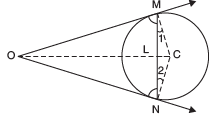Let NM be a chord of a circle with centre C.
Let the tangents at M and N meet at O.
∵ OM is a tangent at M

∵ ∠OMC = 90° ...(1)
Similarly ∠ONC = 90° ...(2)
Since, CM = CN [Radii of the same circle]
∵ In D CMN, ∠1= ∠2
From (1) and (2), we have
∠OMC –∠1 = ∠ONC –∠2
⇒ ∠OML = ∠ONL

Thus, tangents make equal angles with the chord.

Q2. Two concentric circles have a common centre O. The chord AB to the bigger circle touches the smaller circle at P. If OP = 3 cm and AB = 8 cm then find the radius of the bigger circle.

Sol. ∵ AB touches the smaller circle at P.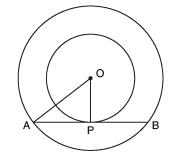∴ OP ⊥ AB ⇒ ∠OPA = 90°
Now, AB is a chord of the bigger circle.
Since, the perpendicular from the centre to a chord, bisects the chord,
∴ P is the mid-point of AB

⇒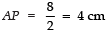In right ∆ APO, we have
AO= OP2 + AP2
⇒ AO2 = 32 + 42
⇒ AO2 = 9 + 16 = 25 = 52

⇒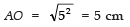Thus, the radius of the bigger circle is 5 cm.

Q3. In the given figure, O is the centre of the circle and PQ is a tangent to it. If its circumference is 12π cm, then find the length of the tangent.

Sol. ∵ Circumference of the circle = 12π cm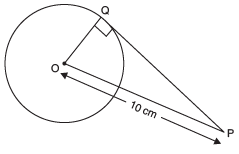∴ 2π r =12π
[∵ r is the radius of the circle]

⇒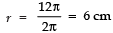⇒ Radius of the circle = 6 cm = OQ

Since a tangent to circle is perpendicular to the radius through the point of contact,
∴ ∠OQP = 90°
Now, in rt Δ OQP, we have:
OQ2 + QP2 = OP2
⇒ 62 + QP2 =102
⇒ QP2 = 102 − 62 = (10 − 6) (10 + 6) = 4 × 16 = 64 = 82

⇒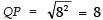Thus, the length of the tangent is 8 cm.

Q4. Given two concentric circles of radii 10 cm and 6 cm. Find the length of the chord of the larger circle which touches the other circle.

Sol. The chord AB touches the inner circle at P.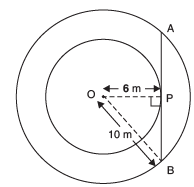∴ AB is tangent to the inner circle.
⇒ OP ⊥ AB

[∵ O is the centre and OP is radius through the point of contact P]
∴ ∠OPB = 90°.
Now, in right ∆ OPB, we have:
OP2 + PB2 = OB2
⇒ 62 + PB2 = 102
⇒ PB2 = 102 − 62
= (10 − 6) × (10 + 6)
⇒ PB2 = 4 × 16
⇒ PB2 = 64 = 82

⇒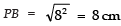∵ The radius perpendicular to a chord bisects the chord.
∴ P is the mid-point of AB
∴ AB = 2 × PB = 2 × 8 = 16 cm.

Q5. Two tangents TP and TQ are drawn to a circle with centre O from an external point T. Prove that ∠PTQ = 2 ∠OPQ.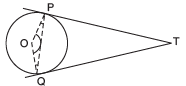Sol. ∵ Tangent to a circle is perpendicular to the radius through the point of contact.
∠OPT + ∠OQT + ∠POQ + ∠PTQ = 360°
or 90° + 90° + ∠POQ + ∠PTQ = 360°
⇒ ∠POQ + ∠PTQ = 360° − 90° − 90° = 180°   ...(1)
In Δ OPQ, ∠1 + ∠2 + ∠POQ = 180°   ...(2)

Since OP =OQ   [Radii of the same circle]
⇒ ∠1 = ∠2    [Angles opposite to equal sides]
∴  ∠OPT = 90° = ∠OQT
∴ From (2), we have
∠1 + ∠1 + ∠POQ = 180°
⇒ 2 ∠1 + ∠POQ = 180° ...(3)
From (1) and (3), we have
2 ∠1 + ∠POQ = ∠POQ + ∠PTQ
⇒ 2 ∠1= ∠PTQ
⇒ 2 ∠OPQ = ∠ PTQ.

Q6. In the figure, the incircle of ∆ ABC touches the sides BC, CA and AB at D, E and F respectively. If AB = AC, prove that BD = CD.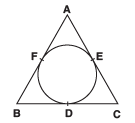Sol. Since the lengths of tangents drawn from an external point to a circle are equal,
∴ We have
AF = AE
BF = BD
CD = CE
(AF + BF) + CD = (AE + CE) + BD
⇒ AB + CD = AC + BD
But AB = AC  (Given)
∴ CD = BD.

Q7. A circle is touching the side BC of a D ABC at P and touching AB and AC produced at Q and R. Prove that: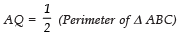Sol. Since, the two tangents drawn to a circle from an external point are equal.
∵  AQ = AR ...(1)
Similarly, BQ = BP ...(2)
and CR = CP ...(3)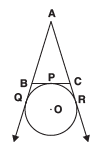Now, Perimeter of Δ ABC
= AB + BC + AC
= AB + (BP + PC) + AC
= AB + (BQ + CR) + AC
[From (2) and (3)]
= (AB + BQ) + (CR + AC)
= AQ + AR
= AQ + AQ [From (1)] = 2AQ
⇒Q8. In the given figure, the radii of two concentric circles are 13 cm and 8 cm. AB is diameter of the bigger circle. BD is the tangent to the smaller circle touching it at D. Find the length AD.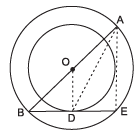Sol. Join AE and OD
∵ ∠ODB = 90°
[BE is a tangent at D and OD is a radius] and ∠AEB = 90° [AB is diameter so ∠AEB is an angle in semicircle so ∠AEB = 90°]
∴ OD ║ ΑΕ and ∆BEA are similar by AA-Similarity

So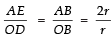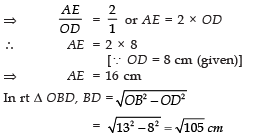Again in rt  Δ AED,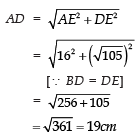Q9. In two concentric circles, a chord of the larger circle touches the smaller circle. If the length of this chord is 8 cm and the diameter of the smaller circle is 6 cm, then find the diameter of the larger circle.

Sol. Let the common centre be O. Let AB be the chord of the larger circle.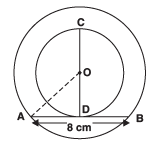∴ AB = 8 cm

And CD is the diameter of the smaller circle i.e., CD = 6 cm

⇒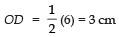Join OA. D is the point of contact.
∴ OD ⊥ AB
⇒ D is the mid point of AB
= 42 + 32 = 16 + 9 = 25 = 52
⇒ AO = 5 cm
⇒ 2AO = 2(5 cm) = 10 cm

∴ The diameter of the bigger circle is 10 cm.

Q10. In the following figure, PA and PB are two tangents drawn to a circle with centre O, from an external point P such that PA = 5 cm and ∠APB = 60°. Find the length of chord AB.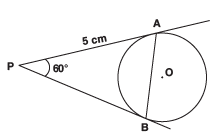Sol. Since the tangents to a circle from an external point are equal,
∴ PA = PB = 5 cm
In ΔPAB, we have
∠PAB = ∠PBA               [∵ PA = PB]
∴∠PAB + ∠PBA + ∠APB = 180°
⇒∠PAB + ∠PAB + 60° = 180°
⇒ 2 ∠PAB + 60° = 180°
⇒ 2 ∠PAB = 180°− 60°
= 120°
⇒ ∠PAB = 60°
⇒ Each angle of ∆PAB is 60°.
⇒ ΔPAB is an equilateral triangle. ∴
PA = PB
= AB = 5 cm
Thus, AB = 5 cm

Q11. In the following figure, AB is a chord of length 9.6 cm of a circle with centre O and radius 6 cm.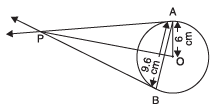The tangents at A and B intersect at P. Find the length PA.

Sol.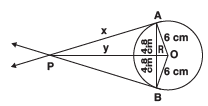Join OB.
Let PA = x cm and PR = y cm Since, OP is perpendicular bisector of AB

∴ AR = BR = 9.6/2 = 4.8 cm

Now, in rt ∆OAR, we have:
OA2 = OR2 + AR2
[By Pythagoras theorem]
⇒ OR2 = OA2 − AR2
= 62 − (4.8)2 = (6 − 4.8) × (6 + 4.8) = 1.2 × 10.8
⇒ = 12.96
OR = 3.6 cm.
Again, in right ΔOAP,
OP2 = AP2 + OA2
OP2 = (AR2 + PR2) + OA2
[∵ AP2 = AR2 + PR2]
⇒ (y + 3.6)2 = (4.8)2 + y2 + 62
⇒y2 + 12.96 + 7.2 y = 23.04 + y2 + 36
⇒ 7.2 y = 46.08

⇒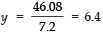⇒ PR = 6.4 cm
Now, AP2 = AP2 + PR2
= (4.8)2 + (6.4)2 = 23.04 + 40.96 = 64

⇒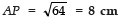Offer running on EduRev: Apply code STAYHOME200 to get INR 200 off on our premium plan EduRev Infinity!

,

,

,

,

,

,

,

,

,

,

,

,

,

,

,

,

,

,

,

,

,

;